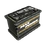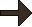# Template:Scrapping table

This template is identical in almost every way to `{{Grid/Crafting Table}}`, except it does not categorize the page using it as a "Craftable item"

## Usage

### Basic usage

```{{Scrapping table
|A1=  |B1=  |C1=  |D1= |E1=
|A2=  |B2=  |C2=  |D2= |E2=
|A3=  |B3=  |C3= CarBattery |D3= |E3=
|A4=  |B4=  |C4=  |D4= |E4=
|A5=  |B5=  |C5=  |D5= |E5=
}}```

### Examples

```{{Scrapping table
|A1=  |B1=  |C1=  |D1= |E1=
|A2=  |B2=  |C2=  |D2= |E2=
|A3=  |B3=  |C3= CarBattery |D3= |E3=
|A4=  |B4=  |C4=  |D4= |E4=
|A5=  |B5=  |C5=  |D5= |E5=